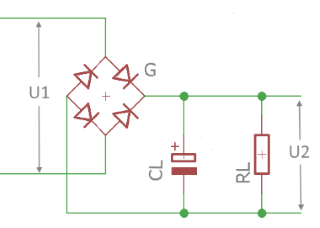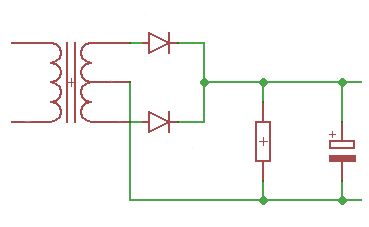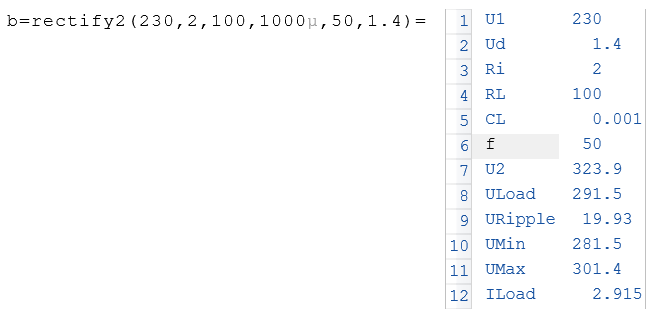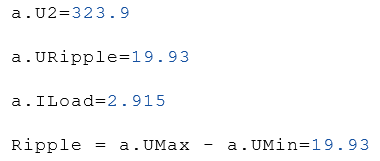# Function Rectify2

Calculates voltages and currents behind a full-wave rectifier

## Description

The function $$Rectify1$$ calculates different voltages and the current behind a Full-wave rectifier with a charging capacitor and a load resistor.

The voltages in idle and under load, the ripple voltage and the current through the load resistor are calculated.

For the internal resistance of the power source, the resistance of the secondary winding of the transformer is essential. In the heavy current range, the resistance of the connection lines can also play a role.

The drop-out voltage at the diode is taken into account. For silicon bridge rectifiers, a value of 1.4 volts for every 2 diodes is common.With full-wave rectification with center tap and two single diodes, 0.7 volts is common.As a result, the function returns an object in which the calculated values are contained.

### Syntax

Rectify2 (U1, Ri, RL, CL, f, UD) = Record

### Symbols of arguments

 U1 RMS value of the alternating voltage in volts before the rectifier Ri Internal resistance of the power source in ohms RL Load resistance in ohms CL Charge consensator in Farads f Frequency of the input voltage in Hz UD Voltage drop of the rectifier diode

### ExampleIf the result is assigned to a variable, the individual values can be accessed via the variable. See the following example.### Results symbols

 U2 Open-circuit DC voltage behind the rectifier ULoad Average DC voltage behind the rectifier under load URipple Superimposed ripple voltage behind the rectifier in Vss UMax Maximum peak voltage after the rectifier UMin Minimum voltage behind the rectifier ILoad Current through the load resistance in A.